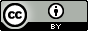# Schultz, T.W.; Bearden, A.P.; Jaworska, J.S. A novel QSAR approach for estimating toxicity of phenols. SAR QSAR Environ. Res. 1996, 5, 2, 99–112.

## QsarDB content

### Property pIGC50: 2-day Tetrahymena toxicity as log(1/IGC50) [log(L/mmol)]

##### 1: All phenols

Regression model (regression)

Open in:QDB Explorer QDB Predictor

Name Type n

R2

σ

Training training 54 0.783 0.363
##### 7: All phenols

Regression model (regression)

Open in:QDB Explorer QDB Predictor

Name Type n

R2

σ

Training training 54 0.843 0.309
##### 8: Selected phenols (pKa >= 9.8)

Regression model (regression)

Open in:QDB Explorer QDB Predictor

Name Type n

R2

σ

Training training 17 0.970 0.130
##### 9: Selected phenols (6.3 <= pKa < 9.8)

Regression model (regression)

Open in:QDB Explorer QDB Predictor

Name Type n

R2

σ

Training training 23 0.799 0.293
##### 11: Selected phenols (pKa < 6.3)

Regression model (regression)

Open in:QDB Explorer QDB Predictor

Name Type n

R2

σ

Training training 14 0.820 0.334

## Citing

When using this QDB archive, please cite (see details) it together with the original article:

 Title: Schultz, T.W.; Bearden, A.P.; Jaworska, J.S. A novel QSAR approach for estimating toxicity of phenols. SAR QSAR Environ. Res. 1996, 5, 2, 99–112. Abstract: Toxicity values (log IGC50(-1)) for 60 phenols tested in the 2-d static population growth inhibition assay with the ciliate Tetrahymena pyriformis were tabulated. Each chemical was selected so the series formed uniform coverage of the hydrophobicity/ionization surface. A high quality hydrophobicity-dependent (log Kow) structure-toxicity relationship (log IGC50(-1) = 0.741 (log Kow)-1.433; n = 17; r2 = 0.970; s = 0.134; F = 486.55; Pr > F = 0.0001) was developed for phenols with pKa values > 9.8. Similarly, separate hydrophobicity-dependent relationships were developed for phenols with pKa values of 4.0, 5.1, 6.3, 7.5, and 8.7. Comparisons of intercepts and slopes, respectively, revealed phenols with pKa values of 6.3 to be the most toxic and the least influenced by hydrophobicity. These relationships were reversed for the more acidic and basic phenols. Plots of toxicity versus pKa for nitro-substituted phenols and phenols with log Kow values of either 1.75 or 2.50 further demonstrated bilinearity between toxicity and ionization. In an effort to more accurately model the relationship between toxicity and ionization, the absolute value function [6.3-pKa] was used to model ionization affects for derivatives with pKa values between 0 and 9.8. For derivatives with pKa value > 9.8, a value of 3.50 was used to quantitate ionization effects. The use of log Kow in conjunction with this modified pKa (delta pKa) resulted in the structure-toxicity relationship (log IGC(50)-1 = 0.567 (log Kow)-0.226 (delta pKa)-0.079; n = 54; r2 = 0.926; s = 0.215; F = 321.06; Pr > F = 0.0001). Derivatives with a nitro group in the 4-position typically did not model well with the above equation. URI: http://hdl.handle.net/10967/71 http://dx.doi.org/10.15152/QDB.71 Date: 2012-05-23
﻿

## Files in this item

Name Description Format Size View
757083443.qdb.zip n/a application/zip 9.302Kb View/OpenFiles associated with this item are distributed